# TFA Discrete Gabor Expansion VI

LabVIEW 2014 Advanced Signal Processing Toolkit Help

Edition Date: June 2014

Part Number: 372656C-01

»View Product InfoDownload Help (Windows Only)

Owning Palette: Time Frequency Transform VIs

Reconstructs time-domain signals from the Gabor elementary functions. Wire data to the signal output to determine the polymorphic instance to use or manually select the instance.

Examples

Use the pull-down menu to select an instance of this VI.

 Select an instance TFA Discrete Gabor Expansion (Waveform)TFA Discrete Gabor Expansion (Real)TFA Discrete Gabor Expansion (Complex)TFA Discrete Gabor Expansion (2D)

## TFA Discrete Gabor Expansion (Waveform)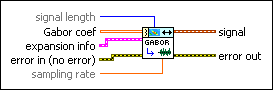signal length specifies the length of the reconstructed signal. The default is –1, which specifies that the length of the reconstructed signal equals M×dM (time steps), where M is the number of rows of Gabor coef.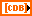Gabor coef specifies the linear time-frequency representation of the signal. Gabor coef contains the weights of the Gabor elementary functions. The element in the ith row and the jth column is the weight of the Gabor elementary function whose time center and frequency center equal i×dt and j×df, respectively, where dt is the time interval between every two contiguous rows of Gabor coef, and df is the frequency interval between every two contiguous columns of Gabor coef. You can use the TFA Coefficients Indicator to display the magnitude of Gabor coef on an intensity graph. You can save the time-dependent 2D array to a text file for use in another software environment. The resulting text file contains only Z values and does not retain the time axis information or the frequency axis information. You can use the TFA Get Time and Freq Scale Info VI to compute the time scale information and the frequency scale information of the time-frequency representation.expansion info specifies the Gabor elementary functions to use for the Gabor expansion.synthesis window specifies the window of the Gabor elementary functions. The size of synthesis window must be divided evenly by dM (time steps) and N (frequency bins).dM (time steps) specifies the time shift, in samples, between elementary functions. The time sampling interval of the signal in the time-frequency domain is dM (time steps)/fs, where fs is the sampling rate of the signal. National Instruments recommends that you set dM (time steps) to a power of 2.N (frequency bins) specifies the number of frequencies of the Gabor elementary functions. The frequency sampling interval of the signal in the time-frequency plane is fs/N (frequency bins), where fs is the sampling rate of the signal. N (frequency bins) must be a power of 2. For stable reconstruction, N (frequency bins) must be greater than or equal to dM (time steps). The ratio between N (frequency bins) and dM (time steps) is called the oversampling rate.error in describes error conditions that occur before this node runs. This input provides standard error in functionality.sampling rate specifies the sampling rate of the reconstructed signal in hertz. sampling rate must be greater than 0, or this VI sets sampling rate to 1 automatically.signal returns the reconstructed signal.error out contains error information. This output provides standard error out functionality.

## TFA Discrete Gabor Expansion (Real)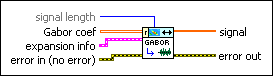signal length specifies the length of the reconstructed signal. The default is –1, which specifies that the length of the reconstructed signal equals M×dM (time steps), where M is the number of rows of Gabor coef.Gabor coef specifies the linear time-frequency representation of the signal. Gabor coef contains the weights of the Gabor elementary functions. The element in the ith row and the jth column is the weight of the Gabor elementary function whose time center and frequency center equal i×dt and j×df, respectively, where dt is the time interval between every two contiguous rows of Gabor coef, and df is the frequency interval between every two contiguous columns of Gabor coef. You can use the TFA Coefficients Indicator to display the magnitude of Gabor coef on an intensity graph. You can save the time-dependent 2D array to a text file for use in another software environment. The resulting text file contains only Z values and does not retain the time axis information or the frequency axis information. You can use the TFA Get Time and Freq Scale Info VI to compute the time scale information and the frequency scale information of the time-frequency representation.expansion info specifies the Gabor elementary functions to use for the Gabor expansion.synthesis window specifies the window of the Gabor elementary functions. The size of synthesis window must be divided evenly by dM (time steps) and N (frequency bins).dM (time steps) specifies the time shift, in samples, between elementary functions. The time sampling interval of the signal in the time-frequency domain is dM (time steps)/fs, where fs is the sampling rate of the signal. National Instruments recommends that you set dM (time steps) to a power of 2.N (frequency bins) specifies the number of frequencies of the Gabor elementary functions. The frequency sampling interval of the signal in the time-frequency plane is fs/N (frequency bins), where fs is the sampling rate of the signal. N (frequency bins) must be a power of 2. For stable reconstruction, N (frequency bins) must be greater than or equal to dM (time steps). The ratio between N (frequency bins) and dM (time steps) is called the oversampling rate.error in describes error conditions that occur before this node runs. This input provides standard error in functionality.signal returns the reconstructed signal.error out contains error information. This output provides standard error out functionality.

## TFA Discrete Gabor Expansion (Complex)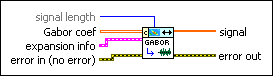signal length specifies the length of the reconstructed signal. The default is –1, which specifies that the length of the reconstructed signal equals M×dM (time steps), where M is the number of rows of Gabor coef.Gabor coef specifies the linear time-frequency representation of the signal. Gabor coef contains the weights of the Gabor elementary functions. The element in the ith row and the jth column is the weight of the Gabor elementary function whose time center and frequency center equal i×dt and j×df, respectively, where dt is the time interval between every two contiguous rows of Gabor coef, and df is the frequency interval between every two contiguous columns of Gabor coef. You can use the TFA Coefficients Indicator to display the magnitude of Gabor coef on an intensity graph. You can save the time-dependent 2D array to a text file for use in another software environment. The resulting text file contains only Z values and does not retain the time axis information or the frequency axis information. You can use the TFA Get Time and Freq Scale Info VI to compute the time scale information and the frequency scale information of the time-frequency representation.expansion info specifies the Gabor elementary functions to use for the Gabor expansion.synthesis window specifies the window of the Gabor elementary functions. The size of synthesis window must be divided evenly by dM (time steps) and N (frequency bins).dM (time steps) specifies the time shift, in samples, between elementary functions. The time sampling interval of the signal in the time-frequency domain is dM (time steps)/fs, where fs is the sampling rate of the signal. National Instruments recommends that you set dM (time steps) to a power of 2.N (frequency bins) specifies the number of frequencies of the Gabor elementary functions. The frequency sampling interval of the signal in the time-frequency plane is fs/N (frequency bins), where fs is the sampling rate of the signal. N (frequency bins) must be a power of 2. For stable reconstruction, N (frequency bins) must be greater than or equal to dM (time steps). The ratio between N (frequency bins) and dM (time steps) is called the oversampling rate.error in describes error conditions that occur before this node runs. This input provides standard error in functionality.signal returns the reconstructed signal.error out contains error information. This output provides standard error out functionality.

## TFA Discrete Gabor Expansion (2D)Note  Reconstructs the 2D signal from Gabor coef.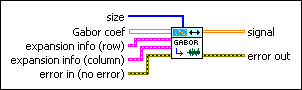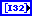size specifies the size of the reconstructed 2D signal as the number of rows followed by the number of columns.Gabor coef specifies the result of the 2D discrete Gabor transform.expansion info (row) specifies the Gabor elementary functions for the row reconstruction.synthesis window specifies the window of the Gabor elementary functions. The size of synthesis window must be divided evenly by dM (time steps) and N (frequency bins).dM (time steps) specifies the time shift, in samples, between elementary functions. The time sampling interval of the signal in the time-frequency domain is dM (time steps)/fs, where fs is the sampling rate of the signal. National Instruments recommends that you set dM (time steps) to a power of 2.N (frequency bins) specifies the number of frequencies of the Gabor elementary functions. The frequency sampling interval of the signal in the time-frequency plane is fs/N (frequency bins), where fs is the sampling rate of the signal. N (frequency bins) must be a power of 2. For stable reconstruction, N (frequency bins) must be greater than or equal to dM (time steps). The ratio between N (frequency bins) and dM (time steps) is called the oversampling rate.expansion info (column) specifies the Gabor elementary functions for the column reconstruction.synthesis window specifies the window of the Gabor elementary functions. The size of synthesis window must be divided evenly by dM (time steps) and N (frequency bins).dM (time steps) specifies the time shift, in samples, between elementary functions. The time sampling interval of the signal in the time-frequency domain is dM (time steps)/fs, where fs is the sampling rate of the signal. National Instruments recommends that you set dM (time steps) to a power of 2.N (frequency bins) specifies the number of frequencies of the Gabor elementary functions. The frequency sampling interval of the signal in the time-frequency plane is fs/N (frequency bins), where fs is the sampling rate of the signal. N (frequency bins) must be a power of 2. For stable reconstruction, N (frequency bins) must be greater than or equal to dM (time steps). The ratio between N (frequency bins) and dM (time steps) is called the oversampling rate.error in describes error conditions that occur before this node runs. This input provides standard error in functionality.signal returns the reconstructed signal.error out contains error information. This output provides standard error out functionality.

## Examples

Refer to the following VIs for examples of using the TFA Discrete Gabor Expansion VI:

• Gabor Transform and Expansion VI: labview\examples\Time Frequency Analysis\TFAFunctions
• Dual Function VI: labview\examples\Time Frequency Analysis\TFAGettingStarted
• Linear JTFA Method VI: labview\examples\Time Frequency Analysis\TFAGettingStarted